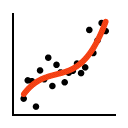#### File Exchange > Graphing >    2D SmootherAuthor:
OriginLab Technical Support
Date Added:
12/15/2017
Last Update:
6/1/2021
Downloads (90 Days):
8549
Total Ratings:
4
File Size:
630 KB
Average Rating:File Name:
2D Smoother.opx
File Version:
1.30
Minimum Versions:
License:
Free
Summary:

Perform smoothing of XY data plots

Description:

This app lets you perform smoothing of XY data plots on a graph.
The App can operate on a single data plot, or on multiple data plots by internally combining the data.

Installation

Download the file 2D Smoother.opx, and then drag-and-drop onto the Origin workspace. An icon will appear in the Apps Gallery window.

Operation

To launch this app, activate a 2D XY plot with one or more data plots and click on the app icon.
The left panel lists existing plots. You can select one or more plots as input.
If Combine & Sort is ON, data will be combined and sorted before further operation.

There are 3 tabs in the dialog for various operations.
You can add one or more smoothed lines by changing settings and clicking on the Add button.

1. Connected Line
This method simply connects the data points using a chose line style.
Select the line type from the drop-down list:
• Cubic Spline: this option is enabled when there are no more than 900 points and they are strictly in ascending/descrending order.
• B-Spline
• Akima Spline
• Bezier
• Modified Bezier
2. Trendline
This method adds a smoothed line by performing curve fitting of the data points.
Select the fitting type from the drop-down list.
You can display the corresponding equation as a label next to the to the end of the trendline by checking the Add Equation as Label checkbox. Adding R-squared as label is supported as well.
• Linear: Performs linear fitting on the data
$$y=ax+b$$
You can fix the intercept to a particular value by checking the corresponding checkbox.
• Polynomial: Performs polynomial fitting on the data.
The polynomial order can be between 2 and 6.
You can fix the intercept to a particular value by checking the corresponding checkbox.
• Power: Performs apparent fit on the data when Fix Y Offset at Zero is checked:
$$\ln\left(y\right)=\ln\left(a\right) + b\ln\left(x\right)$$
Otherwise
$$y=a+bx^c$$
• Logarithmic: Perform apparent fit on the data when Fix X Shift at Zero is checked:
$$y=a+b\ln\left(x\right)$$
Otherwise
$$y=a+b\ln\left(x+c\right)$$
• Exponential: Perform apparent fit on the data when Fix Y Offset at Zero is checked:
$$\ln\left(y\right)=\ln\left(a\right)+bx$$
Otherwise
$$y=y_0+Ae^{k\left(x-x_0\right)}$$
• Piecewise Linear: Performs 2 or 3 segment linear fit to the data.

3. Smoothed Line
The available smoothing methods are:
• Moving Average
• Savitzky-Golay
• Lowess
• Loess
• Whittaker (Pro)
Note smoothed line works better for a large number of data points.

Updates:

v1.3 Whittaker smoothing (since Origin 2021b. Pro only)
v1.1 Output R-squared value.

Reviews and Comments:
 09/08/2020 luoshuiwuhen good01/18/2019 zhao0722 zhao072212/18/2018 OriginLab Hi ucbechz, Thanks for your suggestion. We will update the App to show R-Square in trendline. 12/18/2018 ucbechz no GUI option to out the R-Square value